# How to Create, Access, and Modify Vector Elements in R ?

• Last Updated : 31 Jul, 2021

In this article, we are going to create, modify and access vector elements in the R Programming language. Vector is a one-dimensional data structure that holds multiple data type elements.

### Creating a vector:

It can be done in these ways:

• Using c() function.
• Using : operator.
• Using seq() function.

Method 1: Using C() function.

The c() function in R Language is used to combine the arguments passed to it. We can create a vector by using c()

Syntax: c(value1,value2,……..,value n)

Where, c stands for combine, values are the input data to the vector.

Example 1: So in this program, we are going to create elements from 1 to 20 and display it

## R

 `# create vector with range``# operator from 1 to 20 elements``vec = ``c``(1:20)``print``(vec)`

Output: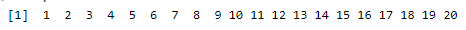Example 2: In this example, we are going to create a vector with different data type elements and display it.

Character type, float type, and int type are used. Int type data is created by using the range operator

## R

 `# create vector with different data type elements``vec=``c``( 1 : 20, ``"sravan"``, ``"deepika"``,``      ``"jyothika"``, 45.7, 56, 34, 56)``print``(vec)`

Output: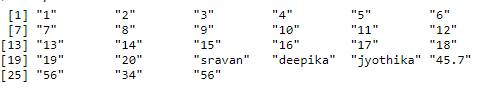Method 2: Using : operator.

We can use : operator to creates the series of numbers in sequence for a vector.

Code:

## R

 `Data <- 1:20;``print``(Data);`

Output:

  1  2  3  4  5  6  7  8  9 10 11 12 13 14 15 16 17 18 19 20

Method 3: Using seq() function.

seq() function is used to create a sequence of elements in a Vector. It takes the length and difference between values as optional argument.

Syntax: seq(from, to, by, length.out)

Parameters:
from: Starting element of the sequence
to: Ending element of the sequence
by: Difference between the elements
length.out: Maximum length of the vector

Code:

## R

 `# R Program to illustrate``# the use of seq() Function``  ` `# Creating vector using seq()``vec1 <- ``seq``(1, 10, by = 2)``  ` `vec2 <- ``seq``(1, 10, length.out = 7)``  ` `# Printing vectors``print``(vec1)``print``(vec2)`

Output:

``` 1 3 5 7 9
  1.0  2.5  4.0  5.5  7.0  8.5 10.0```

### Modifying vector elements

We can modify the vector elements by using [] operator

Syntax: vec[index_value]=new_data

Where index_value is the element index location

Example 1: In this example, we are going to create a vector with 5 integer type elements and modifying the 1st and 2nd elements as 100 and 200

## R

 `# create vector with 5 numbers``vec = ``c``( 10, 20, 3, 4, 5)``print``(vec)` `# change 10 to 100``vec=100` `# change 20 to 200``vec = 200``print``(vec)`

Output: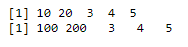Example 2: R program to modify the entire vector at a time.

In this example, we are going to modify the elements from 1 to 20 with 1010 to 120 at a time.

## R

 `# create vector with 20  numbers``vec = ``c``( 1 : 20)``print``(vec)` `# modify all elements at a``# time from (1 t0 20 ) - (101 to 120)``vec[1:20] = 101 : 120``print``(vec)`

Output: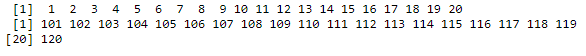### Access vector elements

We can access vector elements using the indexing operator – [] and Indexing starts from 1.

Syntax: vector_name[index_value]

Example 1: R program to display values in a vector

In this example, we are going to create the vector with elements from 100 to 200 using range operator and going to access the 1st, 12th and 13th elements.

## R

 `# create vector with range of``# 100 -200  numbers``vec = ``c``( 100 : 200 )` `# display element 1``print``(vec)` `# display element 12``print``(vec)` `# display element 13``print``(vec)`

Output: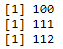Example 2: Display all the elements in a vector

In this example, we created a vector with integer data type elements from 100 to 199, with range operator and display all

## R

 `# create vector with range``# of 100 -200  numbers``vec = ``c``( 100 : 200 )` `# display all``print``(vec[ 1 : 100 ])`

Output: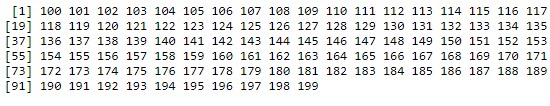My Personal Notes arrow_drop_up